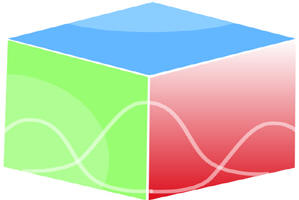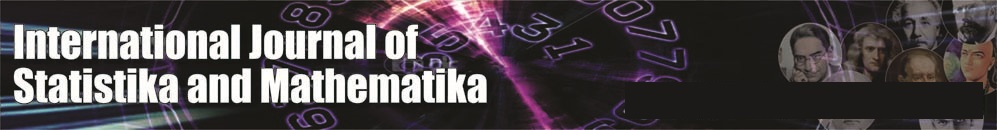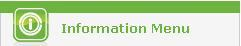``Home| Journals | Statistical Calculator | About Us | Contact UsThe International Journal of Statistika and Mathematika is a refereed stat & math journal devoted to publication of original research articles as well as review articles, with emphasis on unsolved problems and open questions in Statistical & Mathematical sciences. All areas listed on the cover of  Statistical  & Mathematical Reviews, such as stochastic processes, biostatistics, industrial statistics,  pure and applied mathematics, mathematical physics, theoretical mechanics, probability and mathematical statistics, and theoretical biology, are included within the scope of the International Journal of Statistika and MathematikaUntitled Document